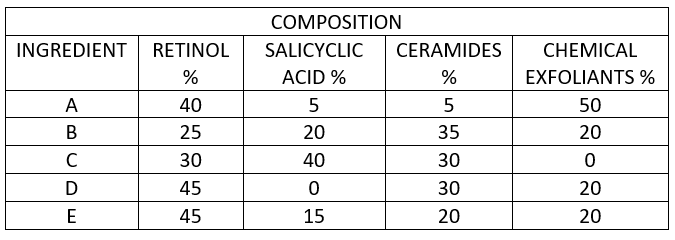60 views

Hindustan Unilever’s R&D is trying to make various formulations for making a new face wash. It is considering 5 ingredients, A, B, C, D and E which can be used in different proportions in the formulations. The cost per unit of the ingredients A, B, C, D and E are 250, 150, 120, 180, 40 respectively.
The table below is the composition of these ingredients.If D and E are mixed in such proportion that the amount of salicylic acid is exactly 3% of the mixture, then the percentage of ceramides in this mixture is?

Let x be the amount of D in the mixture.

(1-x)*15= 3

1-x =.2

x=0.8

Percentage of ceramides = 30*.8+ 20*.2

= 28.

(Q-1) If it is advised that chemical exfoliants and ceramides should be minimum 30% and 20% respectively. In how many different ways can we prepare this formula mixing exactly two ingredients in same quantity?

(Q-2) Which of the following mixtures of two ingredients will result in the lowest cost incurred such that there is atleast 30% of retinol and 10% of Salicyclic acid.

1 vote
1
446 views
2
345 views